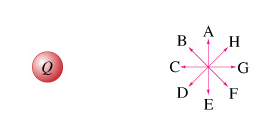# Problem: The total positive charge is Q = 1.62 × 10-6 C.What is the direction of the electric field at point P? (Figure 2)Enter the letter of the vector that represents the direction of EP?

###### FREE Expert Solution

The electric field due to a positive charge is directed radially outward.

90% (362 ratings)###### Problem Details

The total positive charge is Q = 1.62 × 10-6 C.

What is the direction of the electric field at point P? (Figure 2)

Enter the letter of the vector that represents the direction of EP?